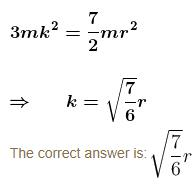# Rigid Body Dynamics MCQ Level - 1

## 10 Questions MCQ Test Topic wise Tests for IIT JAM Physics | Rigid Body Dynamics MCQ Level - 1

Description
This mock test of Rigid Body Dynamics MCQ Level - 1 for Physics helps you for every Physics entrance exam. This contains 10 Multiple Choice Questions for Physics Rigid Body Dynamics MCQ Level - 1 (mcq) to study with solutions a complete question bank. The solved questions answers in this Rigid Body Dynamics MCQ Level - 1 quiz give you a good mix of easy questions and tough questions. Physics students definitely take this Rigid Body Dynamics MCQ Level - 1 exercise for a better result in the exam. You can find other Rigid Body Dynamics MCQ Level - 1 extra questions, long questions & short questions for Physics on EduRev as well by searching above.
QUESTION: 1

### A uniform rod of length L and weight WR is suspended as shown in the accompanying figure. A weights W is added to the end of the rod. The rod rests in horizontal position and is hinged at O. The support wire is at an angle θ to the rod. What is the tension T in the wire?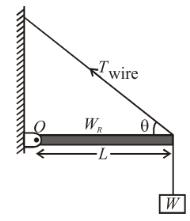Solution:

The forces on rod hinged at O, which cause torque about O are as shown.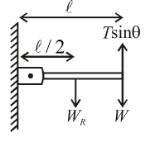Since net torque on rod about hinge at O is zero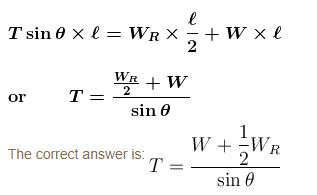QUESTION: 2

### Moment of inertia of a disc object OO' is :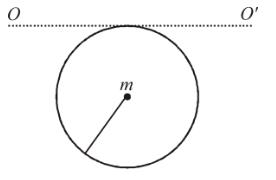Solution: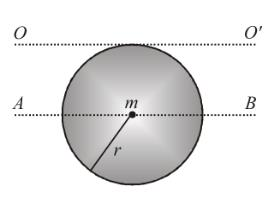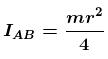(by perpendicular axis theorem)

by applying parallel axis theorem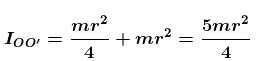The correct answer is: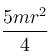QUESTION: 3

### A uniform circular disc placed on a horizontal rough surface has initially a velocity v0 and an angular velocity ω0 as shown in the figure :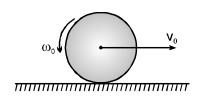The disc comes to rest after moving some distance in the direction of motion. Then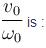Solution: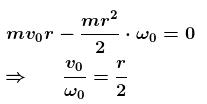QUESTION: 4

The moment of inertia of a door of mass m, length 2ℓ and width ℓ about its longer side is :

Solution: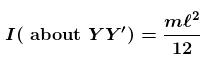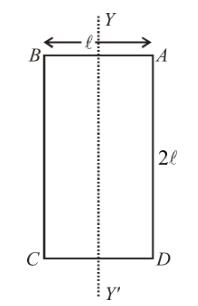Using parallel axis theorem : IAD = ICM + ML2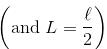I (about AD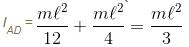The correct answer is: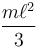QUESTION: 5

Three identical uniform rods, each of length ℓ, are joined to form a rigid equilateral triangle. Its radius of gyration about an axis passing through a corner and perpendicular to the plane of the triangle is :

Solution:

MI of the system w.r.t. an axis ⊥ to plane and passing through one corner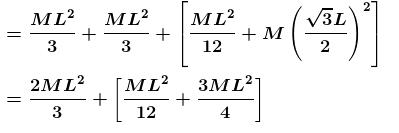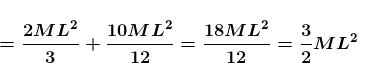Now, the radius of gyration is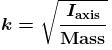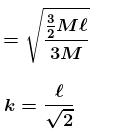The correct answer is: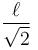QUESTION: 6

A wheel is rolling uniformly along a level road (see fig.) The speed of translational motion of the wheel axis is v. What are the speed of the points A and B on the wheel rim relative to the road at the instant shown in the fig?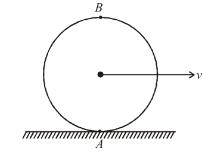Solution: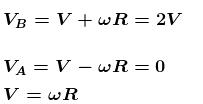The correct answer is: VA = 0, VB = 2V

QUESTION: 7

A uniform cube of side a and mass m rests on a rough horizontal table. A horizontal force mn is applied normal to one of the faces at a point that is directly below the centre of face, at a height 3a/4 above the base. The minimum value of F for which the cube begins to tilt about the edge is? (Assume that the cube does not slide).

Solution:

Taking moments abut point O :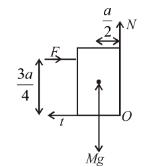Moment of N (normal reaction) and f (force of friction) are zero. In critical case normal will pass through O. To tip about the edge, moment of F should be greater than moment of mg or,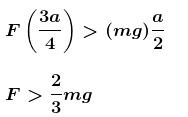The correct answer is: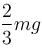QUESTION: 8

A thin wire of length L and uniform density ρ is bent into a circular loop with centre at O as shown. The moment of inertia of the loop about the axis  XX' is :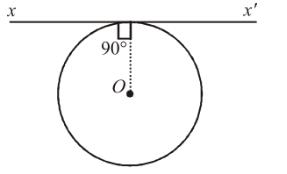Solution: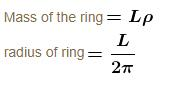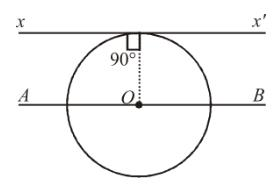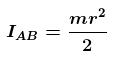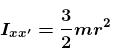(by parallel axis theorem)

by putting value of m and t we get,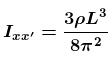The correct answer is: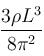QUESTION: 9

A particle performs uniform circular motion with an angular momentum L. If the frequency of particle’s motion is doubled and its kinetic energy is halved, the angular momentum becomes :

Solution:

Kinetic energy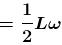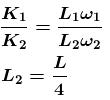QUESTION: 10

Three rings each of mass m and radius r are so placed that they touch each other. The radius of gyration of the system about the axis as shown in the figure is :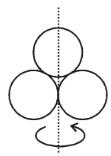Solution:

I = I1 + I2 + I3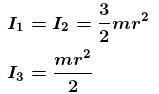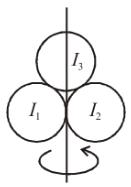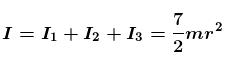Moment of inertia = 3mk2  where k is radius of gyration.